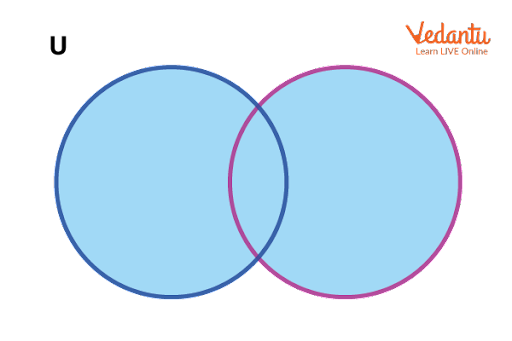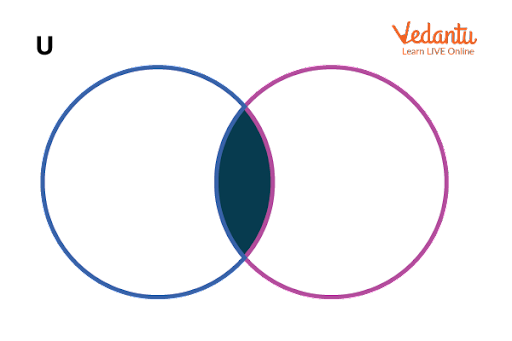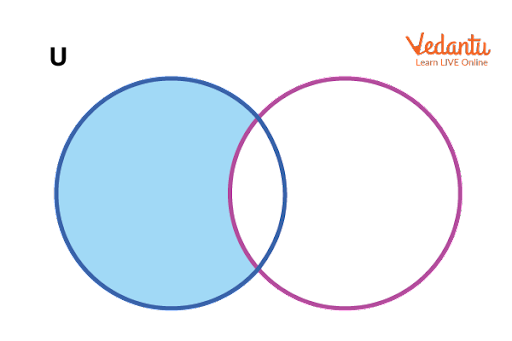Courses
Courses for Kids
Free study material
Offline Centres
More

# Union, Intersection and Difference of Sets for JEELast updated date: 26th Nov 2023
Total views: 21.3k
Views today: 1.21k## A Dive Into the History of Sets

Sets are the well ordered collection of elements such as numbers, variables, symbols, letters, sentences and so forth. Any object that is distinct, and up to our imagination, can be considered an element of the set. The number of elements inside the set is known as the cardinal number of a set. Generally, a set is represented by a capital letter. For example, L = {35, 89, 104} is a set that contains three elements.

Primarily, sets are divided into many types based on the cardinal number, i.e., based on the number of elements inside the set. If a set consists of only one element, then it is called a Singleton set. If there are no elements, then it is an Empty set. Also, if the number of elements is finite, it could be stated as a Finite set. If not, then it is an Infinite set.

There are still many sets defined on the basis of many other properties. To understand those, let us first discuss the Union, Intersection, and Difference of Sets, which are some of the operations performed on two or more sets to produce a single set.

## What is The Union of Sets?

Let us assume there are two sets L and M and the union of these two sets is represented as \$L\cup M\$.

\$L\cup M\$ is the union of two sets L and M which contain all the elements of L and M.

Note: Elements must be distinct and can not be repeated in the Union Set. Every element is such that it may belong to set L or set M, but it must belong to one of those sets.

The union of sets is commutative, i.e., \$L\cup M=M\cup L\$

Let’s comprehend this concept of the union of sets using an example.

Let L = {b, e, i, m, r, w, z} and M = {2, 5, 7, 9, 11, 13, e, r, x, y, z}

Now, \$L\cup M\$ = {b, e, i, m, r, w, z, 2, 5, 7, 9, 11, 13, x, y}

As you can see here, elements that belong to both L and M are written but they are not repeated. This can be shown in a diagram which needs a Universal set U.

Note: A universal set is a set that consists of all the elements related to the described sets in the question given. Suppose if we are asked to describe a set of integers as the union of two sets, the universal set consists of all the numbers like real, complex, integers, rational or irrational including natural. Out of the numbers which are present in the universal set, we make the union taking only integers out of it.Image: Union of two sets L and M

In the above image, the blue-coloured circle is the L set and the pink one is the M set. The blue shaded portion is the union of these two sets L and M.

If there are n number of sets namely, L1, L2, L3 and so on up to Ln then the sets formula for the union if sets are given by the equation.

• \$\Sigma_{i=1}^{n}{L_i}=L_{1} \cup L_{2} \cup L_{3}-------L_{n}\$

## Intersection of Sets

Let L and M be the two sets. The intersection set of L and M is demonstrated as

\$L\cap M\$ and this set consist of elements which are part of both L and M.

That is, if an element is a part of the intersection set \$L\cap M\$, then it must belong to both the L set and the M set.

The intersection is also commutative, that is \$L\cap M=M\cap L\$

Suppose L = {12,27,21,35,86,91,97} and M = {2,20,21,47,54,77,86,97}. Now the intersection set, \$L\cap M\$ = {21,86,97} as only these three elements are in common.

The diagram for the intersection of sets is shown below.Image: Intersection of two sets L and M

In the above image, we can see the part highlighted is the intersection part of the two circles. If there are n numbers of sets, namely L1, L2, L3 and so on up to Ln, then the sets formula for the intersection of these sets is given by

• \$\Sigma_{i=1}^{n}{L_i}=L_{1} \cap L_{2} \cap L_{3}-------L_{n}\$

This shows that the intersection set for the n sets consists of elements that are present in all the n sets.

## Difference Between Sets

If the difference between two sets L and M is L – M, then this set contains the elements which are present in only L but not in M. This is basically removing the elements in the intersection set \$L\cap M\$ from the whole set of L. This gives us the difference between two sets L and M.

Let us understand how this works through a figure.Image: Difference between sets L and M

As shown here, the highlighted part is the section of only L but not M. This is the difference set, L-M.

Note: The difference set is not commutative, i.e., L – M is not equal to M – L.

Let’s have a look at some of the formulas of sets that are useful in solving the problems.

• \$n(L)-n(L \cap M)=n(L-M)\$

• \$n(L \cup M)=n(L)+n(M)-n(L \cap M)\$

• \$n(L-M)+n(L \cap M)+n(M-L)=n(L \cup M)\$

## Conclusion

Therefore, the union of two sets \$L\cup M\$ can be written as the sum of the difference set L – M and the Intersection set \$L\cap M\$ and the difference set M – L. These formulae are proven and the properties of sets are well defined. The union set and the intersection set satisfy the commutative property, associative property and distributive property.

Hence, the questions like what is an intersection and what is a union of sets get clear through this article. The examples of sets discussed are clear and vivid.